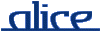# alice library manual.# The PRIM_IO signature

## ________ Synopsis ____________________________________________________

```    signature PRIM_IO
structure BinPrimIO : PRIM_IO where type elem         = Word8.t
and type vector       = Word8Vector.t
and type array        = Word8Array.t
and type vector_slice = Word8VectorSlice.t
and type array_slice  = Word8ArraySlice.t
and type pos          = Position.t
structure TextPrimIO : PRIM_IO where type elem        = Char.t
and type vector       = CharVector.t
and type array        = CharArray.t
and type vector_slice = CharVectorSlice.t
and type array_slice  = CharArraySlice.t
functor PrimIO (structure Vector : MONO_VECTOR
structure Array :  MONO_ARRAY
where type elem   = Vector.elem
and type vector = Vector.t
structure VectorSlice : MONO_VECTOR_SLICE
where type elem   = Vector.elem
and type vector = Vector.t
structure ArraySlice : MONO_ARRAY_SLICE
where type elem   = Vector.elem
and type vector = Vector.t
and type array  = Array.t
and type vector_slice = VectorSlice.t
val someElem : Vector.elem
eqtype pos
val compare :  pos * pos -> order) :
PRIM_IO where type elem         = Vector.elem
and type vector       = Vector.t
and type array        = Array.t
and type vector_slice = VectorSlice.t
and type array_slice  = ArraySlice.t
and type pos          = pos
```

The Standard ML Basis' PRIM_IO signature and PrimIO functor.

## ________ Import ______________________________________________________

Imported implicitly.

## ________ Interface ___________________________________________________

```    signature PRIM_IO =
sig
type elem
type vector
type vector_slice
type array
type array_slice

eqtype pos
val compare : pos * pos -> order

{name :       string,
chunkSize :  int,
readVec :    (int -> vector) option,
readArr :    (array_slice -> int) option,
readVecNB :  (int -> vector option) option,
readArrNB :  (array_slice -> int option) option,
block :      (unit -> unit) option,
canInput :   (unit -> bool) option,
avail :      unit -> int option,
getPos :     (unit -> pos) option,
setPos :     (pos -> unit) option,
endPos :     (unit -> pos) option,
verifyPos :  (unit -> pos) option,
close :      unit -> unit,
ioDesc :     OS.IO.iodesc option}

datatype writer = WR of
{name :       string,
chunkSize :  int,
writeVec :   (vector_slice -> int) option,
writeArr :   (array_slice -> int) option,
writeVecNB : (vector_slice -> int option) option,
writeArrNB : (array_slice -> int option) option,
block :      (unit -> unit) option,
canOutput :  (unit -> bool) option,
getPos :     (unit -> pos) option,
setPos :     (pos -> unit) option,
endPos :     (unit -> pos) option,
verifyPos :  (unit -> pos) option,
close :      unit -> unit,
ioDesc :     OS.IO.iodesc option}

val openVector :       vector -> reader
val openVectorListRd : vector list -> reader
val openVectorListWr : unit -> vector list * writer
val nullRd :           unit -> reader
val nullWr :           unit -> writer
val augmentWriter :    writer -> writer
end
```

## ________ Description _________________________________________________

Items not described here are as in the Standard ML Basis' PRIM_IO signature and PrimIO functor.

openVectorListRd l

Creates a reader whose content is the concatenation of the elements from the list l. The list may contain futures, in which case reading will block as soon as the reader has moved to the future position.

openVectorListWr ()

Creates a list future l and a writer. The list behaves as a concurrent stream, that is, whenever data is passed to the writer, l is extended by one element containg the respective data as a vector. When the writer is closed, the list's tail will become nil.